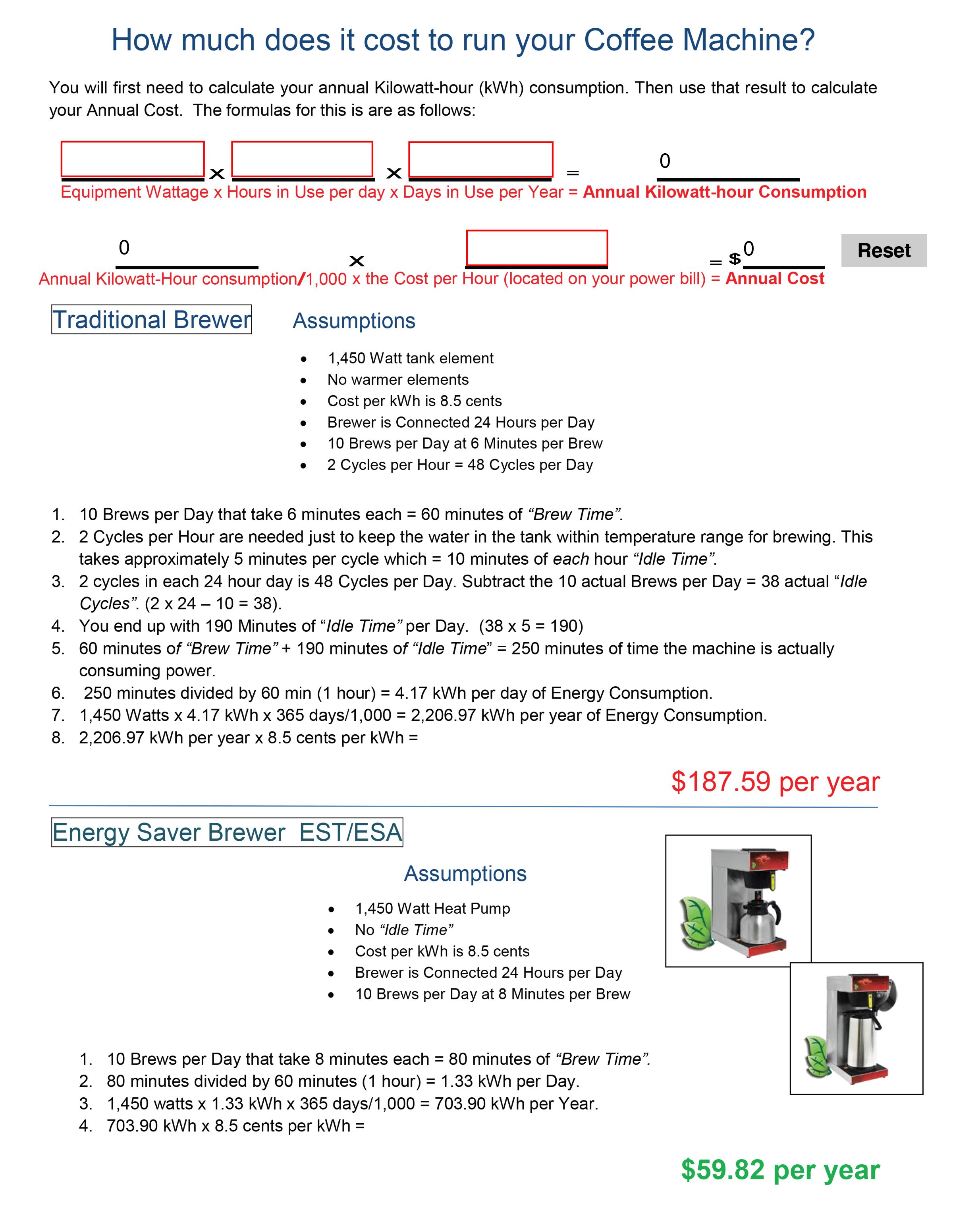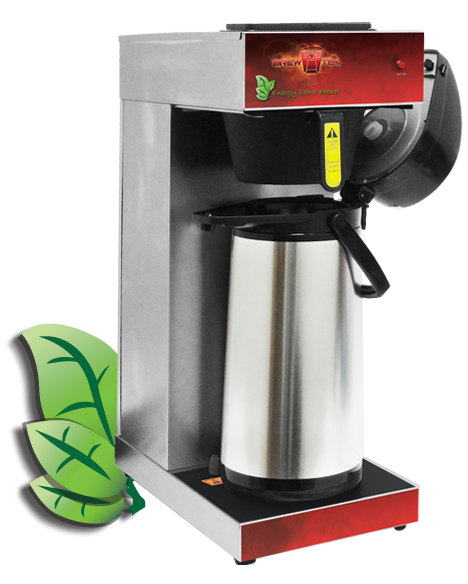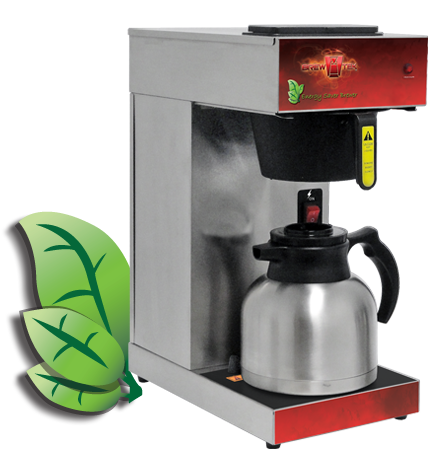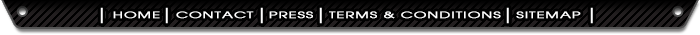How much does it cost to run your Coffee Machine?

You will first need to calculate your annual kilowatt-hour (kWh) consumption. Then use that result to calculate your Annual Cost.Assumptions
• 1,450 watt tank element
• No warmer elements
• Cost per kWh is 8.5 cents
• Brewer is Connected for 24 Hours per Day
• 10 Brews per Day at 6 Minutes per Brew
• 2 cycles per hour less the ten Brews per day = 38 cycles per day
1. 10 Brews per day that take 6 minutes each = 60 minutes of "Brew Time".
2. 2 Cycles per Hour are needed just to keep the water in the tank within temperature range for brewing. This takes approximatley 5 minutes per cycle, which = 10 minutes of each hour "Idle Time".
3. 2 cycles in each 24 hour day is 48 Cycles per Day. Subtract the 10 actual Brews per Day = 38 actual "Idle Cycles". (2 x 24 - 10 = 38).
4. You end up with 190 Minutes of "Idle Time" per Day. (38 x 5 = 190).
5. 60 Minutes of "Brew Time" + 190 Minutes of "Idle Time" = 250 minutes of time the machine is actually consuming power.
6. 250 minutes divided by 60 minutes (1 hour) = 4.17 kWh per day of Energy Consumption.
7. 1,450 Watts x 4.17 kWh x 365 days/1,000 = 2,206.97 kWh per year of Energy Consumption.
8. 2,206.97 kWh per year x 8.5 cents per kWh =
\$187.59 per year

Energy Saver Brewer EST/ESAAssumptions
• 1,450 Watt Heat Pump
• No "Idle Time"
• Cost per kWh is 8.5 cents
• Brewer is Connected for 24 Hours per Day
• 10 Brews per Day at 8 Minutes per Brew
1. 10 Brews per Day that take 8 minutes each = 80 minutes of "Brew Time".
2. 80 minutes divided by 60 minutes (1 hour) = 1.33 kWh per Day
3. 1,450 Watts x 1.33 kWh x 365 days/1,000 = 703.90 kWh per Year.
4. 703.90 kWh per year x 8.5 cents per kWh =
\$59.82 per year